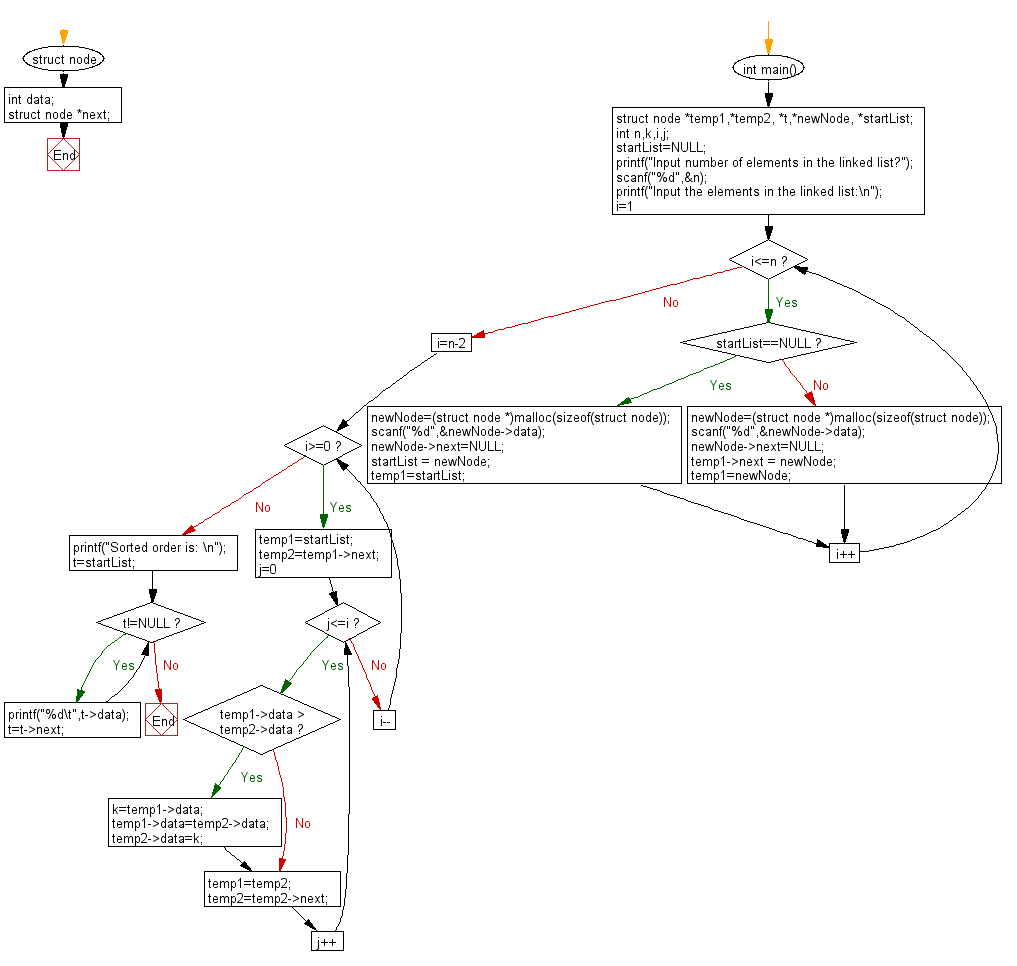﻿ C Program: Sort a given linked list by bubble sort - w3resource

# C Exercises: Sort a given linked list by bubble sort

## C Doubly Linked List : Exercise-20 with Solution

Write a C program to sort a given linked list by bubble sort.

Sample Solution:

C Code:

``````// Licence: https://bit.ly/2JK1psc
#include<stdio.h>
#include <stdlib.h>
struct node
{
int data;
struct node *next;
};
int main()
{
struct node *temp1,*temp2, *t,*newNode, *startList;
int n,k,i,j;
startList=NULL;
printf("Input number of elements in the linked list?");
scanf("%d",&n);
printf("Input the elements in the linked list:\n");
for(i=1;i<=n;i++)
{
if(startList==NULL)
{
newNode=(struct node *)malloc(sizeof(struct node));
scanf("%d",&newNode->data);
newNode->next=NULL;
startList = newNode;
temp1=startList;
}
else
{
newNode=(struct node *)malloc(sizeof(struct node));
scanf("%d",&newNode->data);
newNode->next=NULL;
temp1->next = newNode;
temp1=newNode;
}
}
for(i=n-2;i>=0;i--)
{
temp1=startList;
temp2=temp1->next;
for(j=0;j<=i;j++)
{
if(temp1->data > temp2->data)
{
k=temp1->data;
temp1->data=temp2->data;
temp2->data=k;
}
temp1=temp2;
temp2=temp2->next;
}
}
printf("Sorted order is: \n");
t=startList;
while(t!=NULL)
{
printf("%d\t",t->data);
t=t->next;
}
}
```
```

Sample Output:

``` Input number of elements in the linked list? 5
Input the elements in the linked list: 15
33
49
6
65

Sorted order is:
6	15	33	49	65
```

Flowchart :C Programming Code Editor:

What is the difficulty level of this exercise?

﻿